# Triangle Medians and Centroids

A centroid of a triangle is found at the intersection of the medians of the triangle. A median is a segment drawn from the vertex of a triangle to the midpoint of the opposite side.

## Finding a median

To find the median of a triangle, you first need to find the midpoints of the sides of your triangle. To do this, we will use a simple tool in Geogebra, the Midpoint or Center tool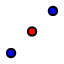. In the exercise below, select the Midpoint or Center tool, which is found under the Point tool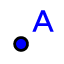. Then simple click on the segment AB. Answer the question below.

What are the approximate coordinates of the midpoint of segment AB?

In the exercise below, find the centroid of the triangle. First find the midpoint of all three sides as you did above. Then connect the midpoints with the opposite vertices. Do this by selecting the Segment tool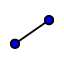, which is found under the Line menu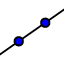. Select the vertex, and then the midpoint that is on the opposite side. After you have constructed all three medians, place a point at their intersection using the Intersect tool. Answer the question that follows.

What are the approximate coordinates of the centroid?

## Special Property of the Centroid

In the exercise below, the centroid (point C) has already been found for you. For this exercise, find the area of each portion of the overall triangle. Each portion (there are 6 total) is shaded a different color. First select the Area tool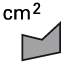, which is found under the Angle menu. Then simply click on each of the individual portions. Answer the questions that follow.

What do you notice about the area of each smaller triangle?

Recall that the area of a shape is the amount of space inside that shape. Based on this, what could we say the centroid is the center of?

Assume that the triangle portions all have a mass (similar to weight) that is distributed evenly across their entire area. Based on this, what could we now say the centroid is the center of? (*Hint, we could also call it the balancing point).